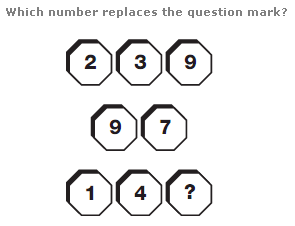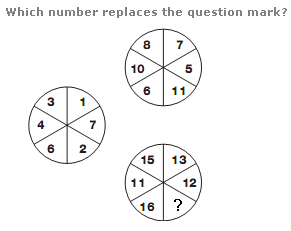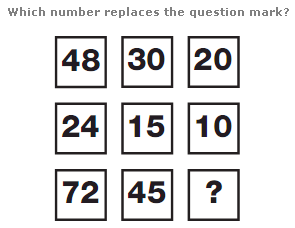# Puzzles - Number puzzles

### Exercise :: Number puzzlesAnswer : 2 Explanation : Reading each row as a 3 or 2 digit number, subtract the central 2 digit value from the upper 3 digit value, to give the 3 digit result on the lower row.Answer : 10 Explanation : Starting with the left hand circle, add 4 to each number and rotate their positions 1 place clockwise to give the values in the upper right hand circle, then add 5 to each of these values and rotate their positions 1 place clockwise to give the values in the lower right hand circle.Answer : 30 Explanation : Working in columns, divide the top value by 2 to give the middle value, and multiply this by 3 to give the bottom value.